Ubuntu

# Bash “If String Equals” ComparisonThe strings in Bash can easily be compared with one another in almost the same manner as we compare the numbers in Bash. There are different operators available in Bash for string comparison, however, as far as this article is concerned, we will learn to use the Bash “if string equals” comparison operator.

## Equality Comparison of the Strings in Bash

To learn the equality comparison of the strings in Bash, you can go through the following three examples:

### Example 1:

In this example, we will simply use the equality comparison operator in Bash to compare the string values contained inside two variables. For that, we use the Bash script shown in the following image: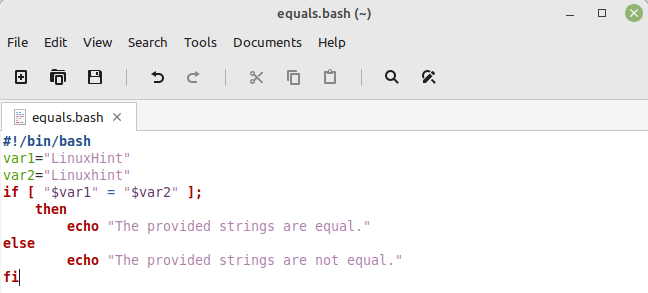In this script, we first declare the two variables and assign them the two different strings with minor differences. Then, we use an “if-else” statement inside which we compare these two variables by using the equality comparison operator. If these strings are equal, the “if” part of the statement is executed. Otherwise, the “else” part is executed.

To run this Bash script, we use the command that follows:

```\$ bash equals.bash
```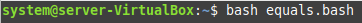The following output implies that the two strings were not equal.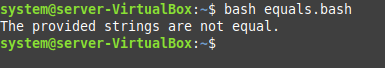### Example 2:

In this example, we will use the equality comparison operator of Bash to compare a variable containing a string with another string. For that, we use the following Bash script: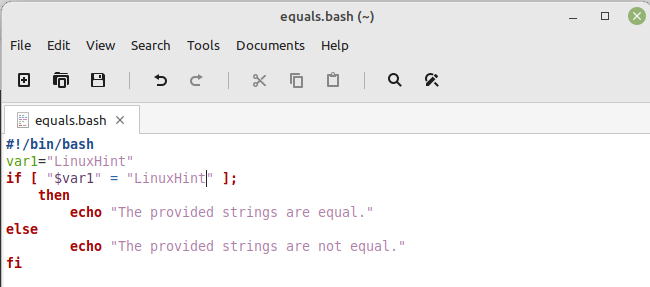In this script, we only define a single variable and assign a string to it. Then, we use an “if-else” statement inside which we compared this variable with a string. If the value of this variable is equal to the provided string, the “if” part of the statement is executed. Otherwise, its “else” part is executed.

When we execute this script, we find out that the two strings were equal as shown in the following image: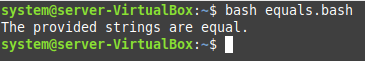### Example 3:

In this example, we will compare our two strings in the same manner as we did in our first example. However, this time, we will use a complete sentence as our string to verify if our method works fine in this situation or not. To do this, we use the following Bash script: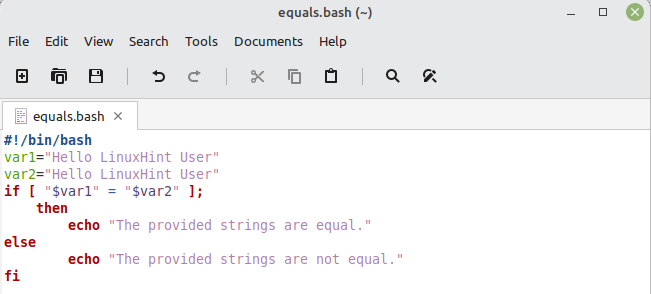In this script, we define the two variables and assign them the two sentences as strings. Then, we simply compare these strings by making use of the equality comparison operator in Bash.

Upon the execution of this script, we figured out that the two provided strings were equal as shown in the following image. This implies that this method works well regardless of the length of the provided string.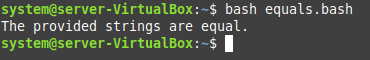## Conclusion

In this article, we discussed about the equality comparison operator for the strings in Bash. For explaining this concept clearly, we demonstrated three different examples to you. Through these examples, you will be able to realize that the strings can be compared in Bash very easily irrespective of their length.